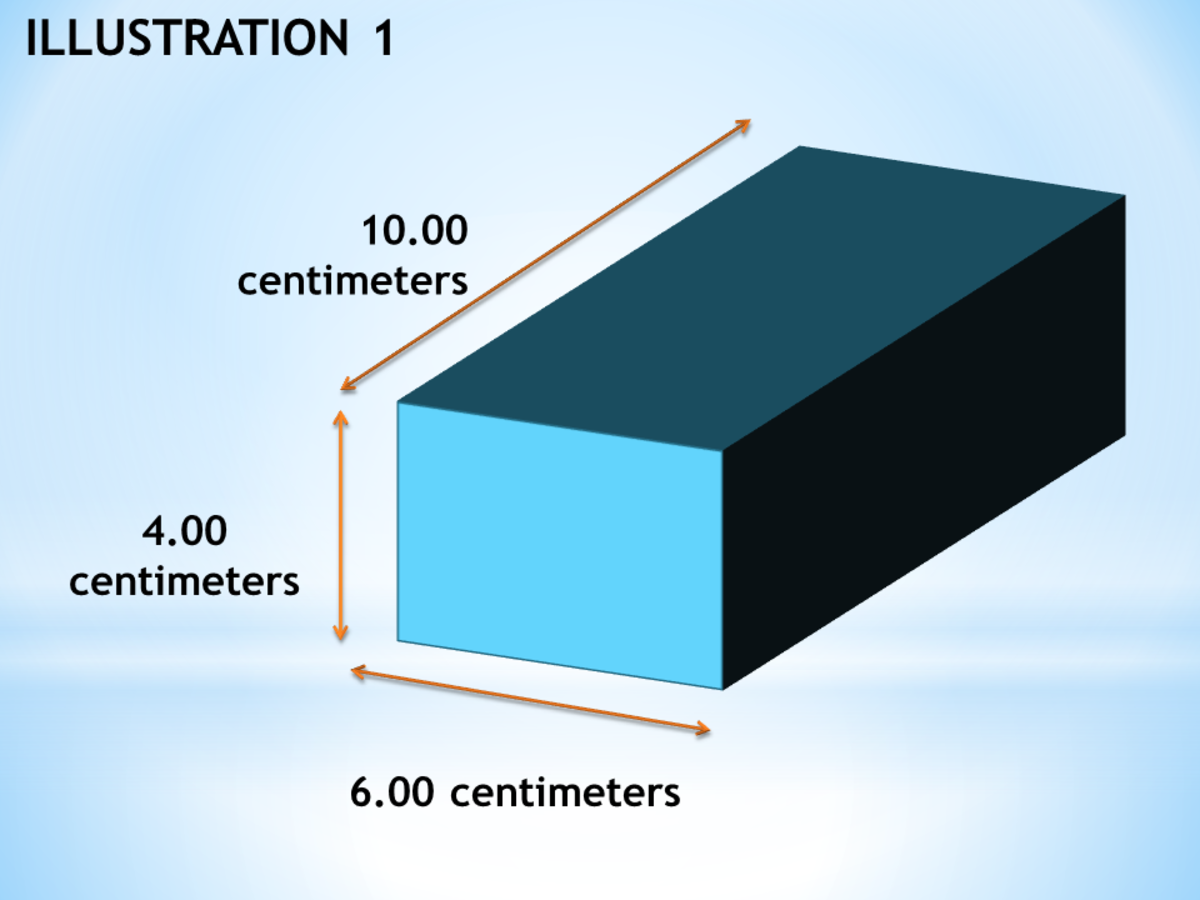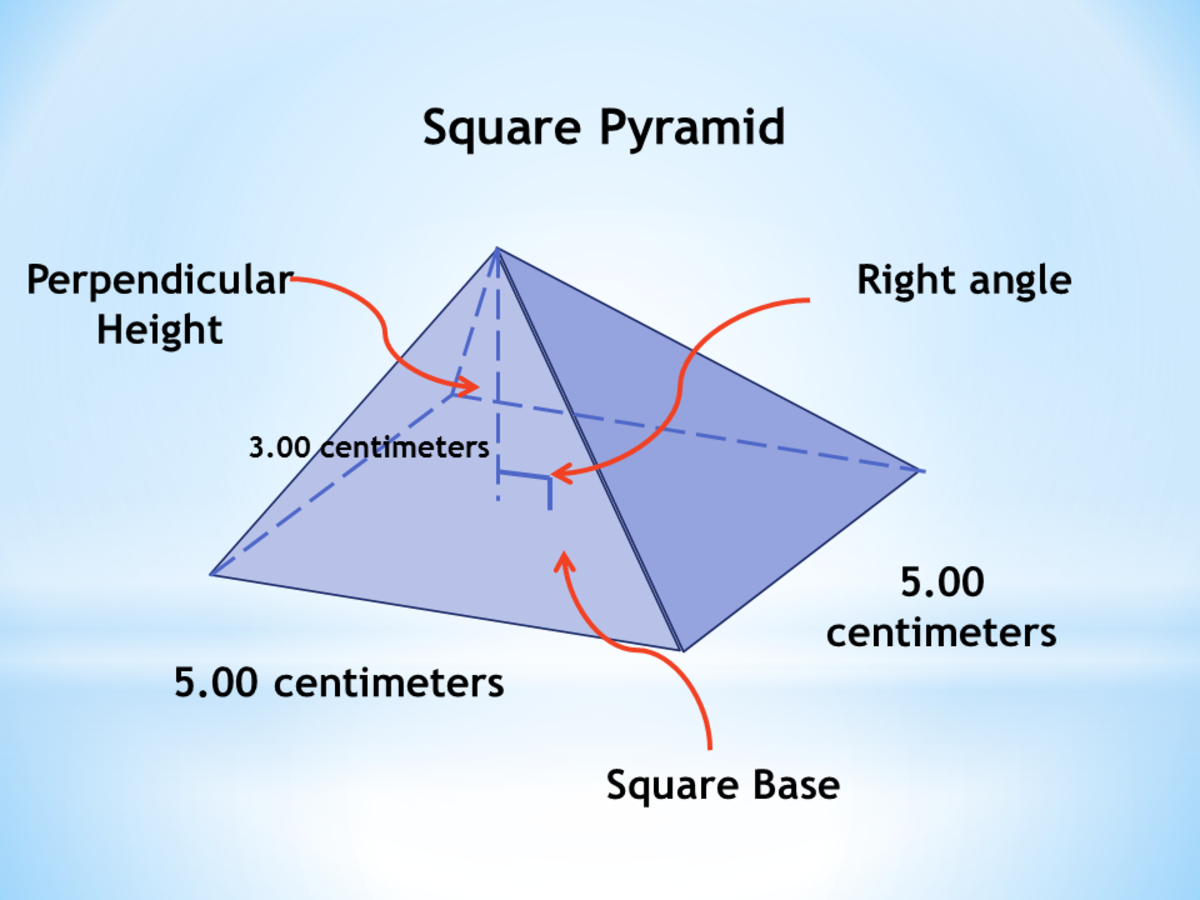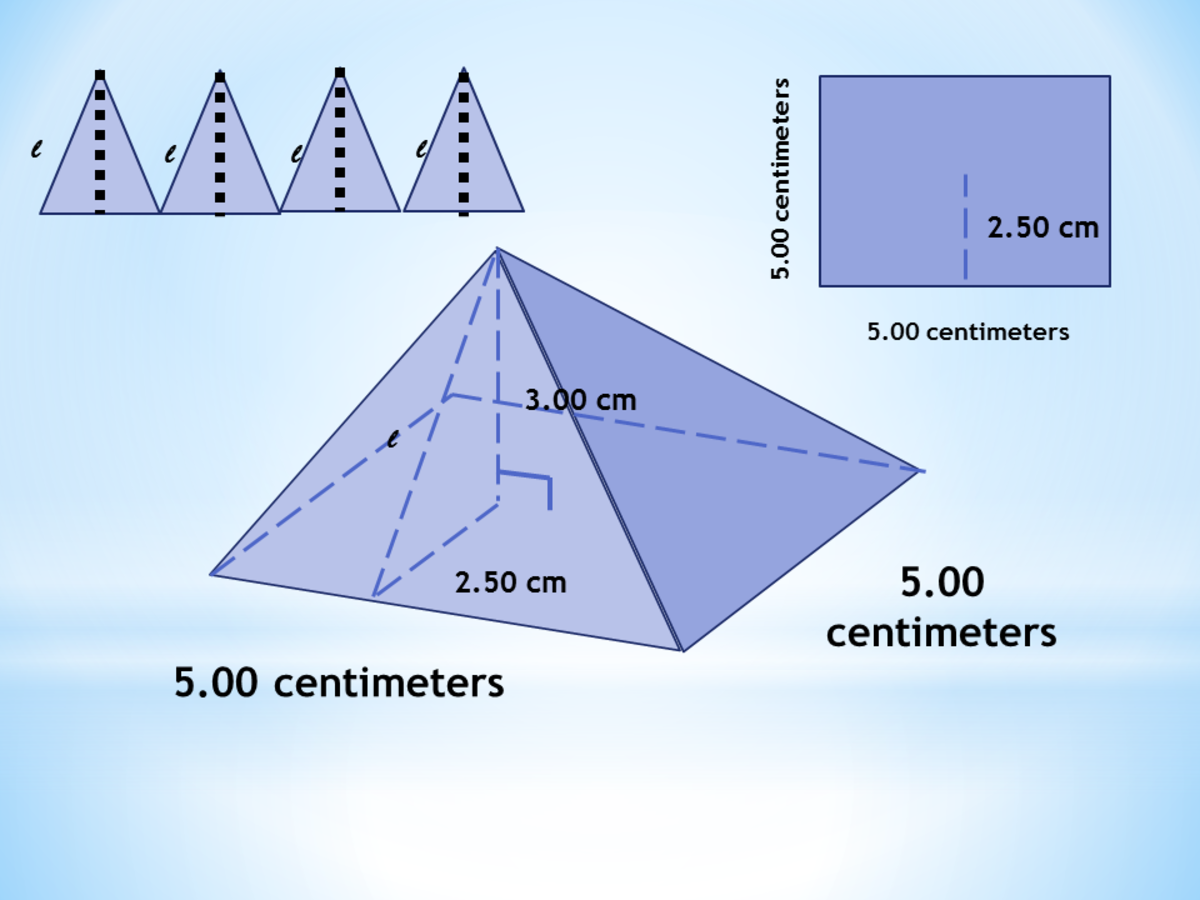# How to Solve for the Surface Area and Volume of Prisms and Pyramids

• Author:
• Updated date:

Ray is a licensed engineer in the Philippines. He loves to write about mathematics and civil engineering.

## What Is a Polyhedron?

A polyhedron is a solid figure formed by different plane surfaces called polygons that enclose a space. A polyhedron has three primary elements: faces, edges, and vertices. The faces of a polyhedron are polygonal surfaces like triangles, squares, hexagons, and more. The segments where two polygonal surfaces join are called the edges, and the segments where two or more sides join are called the vertices.

## Prisms

Prisms are polyhedrons that have two equal parallel polygonal surfaces known as the base. These bases can be in different shapes. The faces connecting the two base sides are parallelograms called lateral faces. The segments where these lateral faces join are called the lateral edges. The crucial element of prisms is height. The height of a prismatic solid is the perpendicular distance between the surfaces of the two bases.

There are different kinds of prisms. There are rectangular prisms, triangular prisms, oblique prisms, pentagonal prisms, and many more. There are two major classes. "Right prisms" are the upright prisms whose lateral faces are rectangles. On the other hand, "oblique prisms" are those whose lateral faces are parallelograms. A prism is named based on the polygonal surfaces of the bases. For example, the polygonal base of a prismatic solid is a rectangle. It is called a rectangular prism because of the polygonal base. The form is [polygonal base] + [prism].

### Surface Area of Prisms

Surface Area means the total area of the polygonal surfaces that make up a polyhedron or solid. It is the summation of all areas including the bases and the lateral faces. Here is the step-by-step procedure for solving for the surface area of any prism.

Step 1: Count the total number of faces. There should be more than five.

Step 2: Identify the dimensions of each face of the prism. As much as possible, draw the exploded view of the faces.

Step 3: Solve for the area of each face of the prism. Multiply the areas by how many faces of equal dimensions there are.

Step 4: Sum up the areas of the faces and bases of the prism.

Prism Surface Area = n (Area 1) + n (Area 2) +...

For a right prism whose base is a regular polygon with 'n' number of sides, 'b' as the length of each side, 'a' as the apothem, and 'h' as the height, the surface area is:

Surface Area = (n x b x a) + (n x b x h)
Surface Area = (n x b) (a + h)

### Volume of Prisms

Volume is the amount of space in a polyhedron or solid. One cubic unit is 1 unit of length, 1 unit of width, and 1 unit of depth. In layman's terms, it is the number of 1 cubic unit cubes that can be stacked to fill up the space of a prism. The formula for the volume of right prisms with a height 'h' is:

Scroll to Continue

Prism Volume = Area of the base (height)

### Example 1: Surface Area and Volume of a Prism

Using the dimensions 4.00 cm x 6.00 cm x 10.00 cm, find the surface area and the volume of the rectangular prism given below.An example illustrating the surface area and volume of a prismJohn Ray Cuevas

Surface Area Solution

The rectangular prism has six faces. The top and bottom polygonal surfaces have dimensions of 6.00 cm x 10.00 cm, the front and back have 4.00 cm x 6.00 cm, and the two sides have 4.00 cm x 10.00 cm. Open up the rectangular prism and explode the faces to have a better view. Lastly, you may now compute for the surface area by adding the area of the surfaces.

Area of top and bottom = 6.00 cm x 10.00 cm

Area of top and bottom = 60.00 square centimeters

Area of front and back = 4.00 cm x 6.00 cm

Area of front and back = 24.00 square centimeters

Area of left and right sides = 4.00 cm x 10.00 cm

Area of left and right sides = 40.00 square centimeters

Prism surface area = 60.00 + 24.00 + 40.00

Prism surface area = 124.00 square centimeters

Volume Solution

Area of the base = 10.00 cm x 6.00 cm

Area of the base = 60.00 square centimeters

Prism Height = 4.00 centimeters

Prism Volume = Area of the base x Height

Prism Volume = 60.00 square centimeters x 4.00 centimeters

Prism Volume = 240.00 cubic centimeters

## Pyramids

A pyramid is a polyhedron with only one base. This base can be of any polygon or shape. The faces of a pyramid intersect at one point called the vertex. One fact about pyramids is that all lateral faces are triangles. Similar to prisms, the height of pyramids is the perpendicular distance from the vertex to the base. A pyramid is named based on the polygonal surfaces of the bases. For example, the polygonal base of a pyramid is a hexagon. It is called a hexagonal pyramid because of the polygonal base. The form is [polygonal base] + [pyramid].

### Surface Area of Pyramids

Surface area means the total area of the polygonal surfaces that make up a polyhedron or solid. It is the summation of all areas including the bases and the lateral faces. Here is the step-by-step procedure in solving for the surface area of any pyramid.

Step 1: Count the total number of triangles. It should be equal to or more than three faces.

Step 2: Identify the dimensions of each face of the pyramid as well as the base. As much as possible draw the exploded view of the faces.

Step 3: Solve for the area of the base of the pyramid.

Step 4: Solve for the area of the triangles. Given the perpendicular height, solve for the slant height.

Step 5: Sum up the areas of the faces and bases of the pyramid.

For pyramids whose base is a regular polygon with 'n' number of sides, 'b' as the length of each side, 'a' as the apothem, and 'l' as the slant height, the surface area is:

Surface Area = (n x b)/2 + (a + l)

### Volume of Pyramids

Volume is the amount of space in a polyhedron or solid. One cubic unit is 1 unit of length, 1 unit of width, and 1 unit of depth. In layman's terms, it is the number of 1 cubic unit cubes that can be stacked to fill up the space of a polyhedron or solid. The formula for the volume pyramids with a height 'h' is:

Pyramid Volume = (1/3) (Area of the base) (height)

### Example 2: Surface Area and Volume of a Pyramid

Find the surface area and volume of the square pyramid shown below.A problem involving the surface area and volume of pyramidJohn Ray Cuevas

Surface Area Solution

A square pyramid has five faces. The surface area of a square pyramid is equal to the sum of the areas of the triangles and the square base. The polygonal base has dimensions 5.00 cm x 5.00 cm.

Base Area = 5.00 cm x 5.00 cm

Base Area = 25.00 square centimeters

Next, calculate for the area of the triangles. In solving the area of the triangles, create a right triangle inside the solid whose hypotenuse is the face of the triangles. Thus, use the Pythagorean theorem to solve for the hypotenuse which is the altitude of the triangles.

l = √(2.50)2 + (3.00)2

l = 3.91 centimeters

Triangular area = 1/2 (5.00 cm) (3.91 cm)

Triangular area = 9.78 square centimeters

Total triangular area = 4 (9.78 square centimeters)

Total triangular area = 39.10 square centimeters

Pyramid surface area = 39.10 square centimeters + 25 square centimeters

Pyramid surface area = 64.10 square centimetersA solution to the surface area of a pyramidJohn Ray Cuevas

Volume Solution

Pyramid Height = 3.00 centimeters

Area of the base = 5.00 cm x 5.00 cm

Area of the base = 25 square centimeters

Pyramid Volume = (1/3) (Area of the base) (height)

Pyramid Volume = (1/3) (25 square centimeters) (3.00 cm)

Pyramid Volume = 25 cubic centimeters

Urvashi Sinha on December 20, 2019:

Thanks so much

Ray (author) from Philippines on June 30, 2018:

Wow, your 8-year old son is really smart. Hope my article helps you in any way. Thanks, Sir Eric!

Eric Dierker from Spring Valley, CA. U.S.A. on June 30, 2018:

We did not study but my son and I started the vocabulary here. Thank you. 8 year olds are smart.